Perpendicular Lines MathBitsNotebook.com Terms of Use   Contact Person: Donna RobertsNOTE: The strategies for proofs of the theorems stated on this page are "discussed" only.
A "formal" proof would require that more details be listed.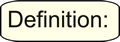Two lines are perpendicular if and only if they form a right angle.
 Perpendicular lines (or segments) actually form four right angles, even if only one of the right angles is marked with a box. The statement above is actually a theorem which is discussed further down on this page.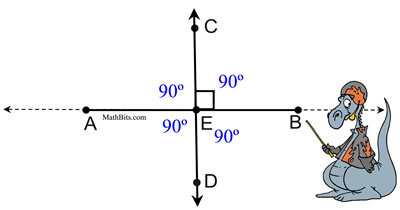There are a couple of common sense concepts relating to perpendicular lines:

 1. The shortest distance from a point to a line is the perpendicular distance. Any distance, other than the perpendicular distance, from point P to line m will become the hypotenuse of the right triangle. It is known that the hypotenuse of a right triangle is the longest side of the triangle.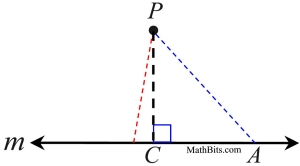2.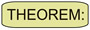In a plane, through a point not on a line, there is one, and only one, perpendicular to the line. If we assume there are two perpendiculars to line m from point P, we will create a triangle containing two right angles (which is not possible). Our assumption of two perpendiculars from point P is not possible.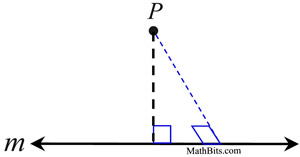Perpendicular lines can also be connected to the concept of parallel lines:

 3.In a plane, if a line is perpendicular to one of two parallel lines, it is also perpendicular to the other line. In the diagram at the right, if m | | n and t ⊥ m, then t ⊥ n. The two marked right angles are corresponding angles for parallel lines, and are therefore congruent. Thus, a right angle also exists where line t intersects line n.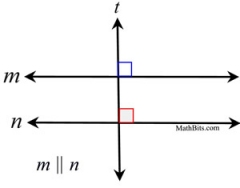4.In a plane, if two lines are perpendicular to the same line, the two lines are parallel. In the diagram at the right, if t ⊥ m and s ⊥ m, then t | | s. Since t and s are each perpendicular to line m, we have two right angles where the intersections occur. Since all right angles are congruent, we have congruent corresponding angles which create parallel lines.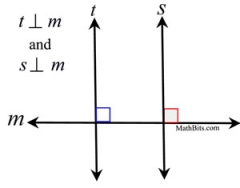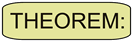If two lines are perpendicular, they form four right angles.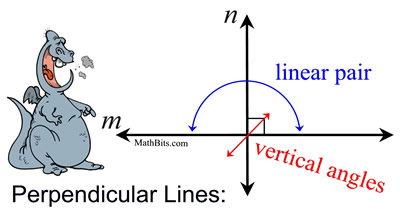When two lines are perpendicular, there are four angles formed at the point of intersection. It makes no difference "where" you label the "box", since all of the angles are right angles. By vertical angles, the two angles across from one another are the same size (both 90º). By using a linear pair, the adjacent angles add to 180º, making any angle adjacent to the box another 90º angle.If two lines intersect to form a linear pair of congruent angles, then the lines are perpendicular.
 When two adjacent angles form a linear pair, their non-shared sides form a straight line (m). This tells us that the measures of the two angles will add to 180º. If these two angles also happen to be congruent (of equal measure), we have two angles of the same size adding to 180º. Each angle will be 90º making m ⊥ n.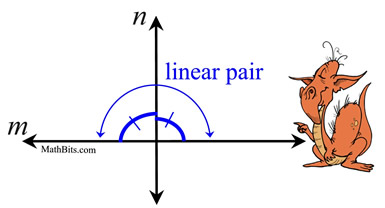If two sides of two adjacent acute angles are perpendicular, then the angels are complementary.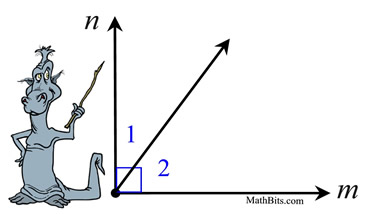In the diagram at the left, <1 and <2 are acute adjacent angles whose non-shared sides are perpendicular, m ⊥ n. Since perpendiculars form right angles (90º), we know the m<1 + m<2 = 90º, making the angles complementary, by definition.